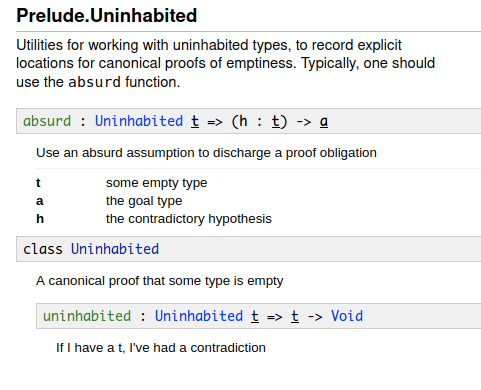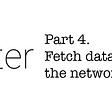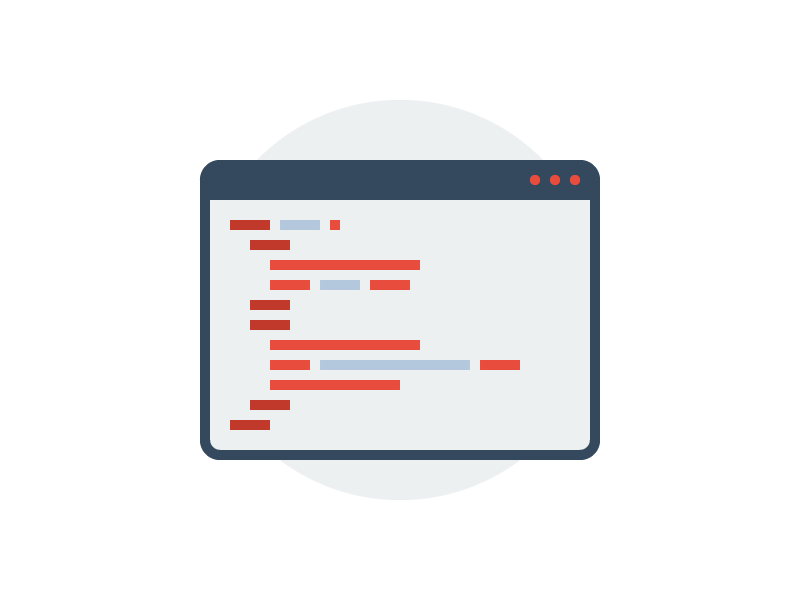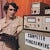# idris proofs: a tale of alists with guaranteed unique values and a bunch of misc

• How to actually use absurd.
• How to use the “No contra” result from DecEq in a with rule.
• How to use Idris’ DPair.
`module Data.AListimport Data.Boolimport Decidable.EqualitykeyInList : Eq a => a -> List (Pair a b) -> BoolkeyInList k [] = FalsekeyInList k ((k1, v1) :: others) =  if k == k1 then    True  else    keyInList k othersallKeysUniqueInList : Eq a => List (Pair a b) -> BoolallKeysUniqueInList [] = TrueallKeysUniqueInList ((k, v) :: others) = let   headIsUnique = not (keyInList k others)   tailsAreUnique = allKeysUniqueInList others in tailsAreUnique && headIsUniquekvCons : a -> b -> List (Pair a b) -> List (Pair a b)kvCons k v l = (k,v) :: lappendBreak : Eq a => (k : a) -> (v : b) -> (l : List (Pair a b)) -> (p : allKeysUniqueInList l = True) -> (DPair (List (Pair a b)) (\l => allKeysUniqueInList l = True))appendBreak k v l p with (decEq (keyInList k l) True)  appendBreak k v l p | Yes prf = (l ** p)  appendBreak k v l p | No contra =    let      cproof = invertContraBool (keyInList k l) True contra    in    (kvCons k v l ** outProof k l p cproof)    where      outProof : (k : a) -> (l : List (Pair a b)) -> (p : allKeysUniqueInList l = True) -> (kp : not (keyInList k l) = True) -> (allKeysUniqueInList l && not (keyInList k l) = True)      outProof k l p kp =        rewrite kp in        rewrite p in        Reflpublic exportproveList : Eq a => (l : List (Pair a b)) -> Maybe (DPair (List (Pair a b)) (\l => allKeysUniqueInList l = True))proveList l with (decEq (allKeysUniqueInList l) True)  proveList l | Yes prf = Just (l ** prf)  proveList l | No _ = Nothingpublic exportaddIfUnique : Eq a => (k : a) -> (v : b) -> (l : DPair (List (Pair a b)) (\l => allKeysUniqueInList l = True)) -> DPair (List (Pair a b)) (\l => allKeysUniqueInList l = True)addIfUnique k v (l ** p) = appendBreak k v l p`
`----------------------------------------- Decidability specialized on bool---------------------------------------||| You can reverse decidability when bool is involved.-- Given a contra on bool equality (a = b) -> Void, produce a proof of the opposite (that (not a) = b)public exportinvertContraBool : (a : Bool) -> (b : Bool) -> (a = b -> Void) -> (not a = b)invertContraBool False False contra = absurd \$ contra ReflinvertContraBool False True contra = ReflinvertContraBool True False contra = ReflinvertContraBool True True contra = absurd \$ contra Refl`Idris’ documentation for Prelude.Uninhabited, including the “absurd” function and Uninhabited typeclass.
`public exportinvertContraBool : (a : Bool) -> (b : Bool) -> (a = b -> Void) -> (not a = b)invertContraBool False False contra = absurd \$ contra Refl`
`public exportinvertContraBool : (a : Bool) -> (b : Bool) -> (a = b -> Void) -> (not a = b)invertContraBool a b contra = invertContraBool_ a b contra  where    fEf : False = False    fEf = Refl    tEt : True = True    tEt = Refl    invertContraBool_ : (a : Bool) -> (b : Bool) -> (a = b -> Void) -> (not a = b)    invertContraBool_ False False contra = absurd (contra fEf)    invertContraBool_ False True contra = Refl    invertContraBool_ True False contra = Refl    invertContraBool_ True True contra = absurd (contra tEt)`
`appendBreak : Eq a => (k : a) -> (v : b) -> (l : List (Pair a b)) -> (p : allKeysUniqueInList l = True) -> (DPair (List (Pair a b)) (\l => allKeysUniqueInList l = True))appendBreak k v l p with (decEq (keyInList k l) True)  appendBreak k v l p | Yes prf = (l ** p)  appendBreak k v l p | No contra =    let      cproof = invertContraBool (keyInList k l) True contra    in    (kvCons k v l ** outProof k l p cproof)    where      outProof : (k : a) -> (l : List (Pair a b)) -> (p : allKeysUniqueInList l = True) -> (kp : not (keyInList k l) = True) -> (allKeysUniqueInList l && not (keyInList k l) = True)      outProof k l p kp =        rewrite kp in        rewrite p in        Refl`
`cproof : not (keyInList k l) = Truecproof = invertContraBool (keyInList k l) True contra`
`outProof : (k : a) -> (l : List (Pair a b)) -> (p : allKeysUniqueInList l = True) -> (kp : not (keyInList k l) = True) -> (allKeysUniqueInList l && not (keyInList k l) = True)      outProof k l p kp =        rewrite kp in        rewrite p in        Refl`
`allKeysUniqueInList ((k, v) :: others) = let   headIsUnique = not (keyInList k others)   tailsAreUnique = allKeysUniqueInList others in tailsAreUnique && headIsUnique`
`proofPair : (v : a) -> (f : a -> Bool) -> (p : f v = True) -> (v ** f v = True)proofPair v f p = MkDPair v p`
`λΠ> :t proofPairData.AList.proofPair : (v : a) -> (f : (a -> Bool)) -> f v = True -> DPair a (\v => f v = True)`
`DPair (List (Pair a b)) (\l => allKeysUniqueInList l = True)`
`addIfUnique k v (l ** p) = appendBreak k v l p -- GoodaddIfUnique k v (MkDPair l p) = appendBreak k v l p-- also good`
`public exportproveList : Eq a => (l : List (Pair a b)) -> Maybe (DPair (List (Pair a b)) (\l => allKeysUniqueInList l = True))proveList l with (decEq (allKeysUniqueInList l) True)  proveList l | Yes prf = Just (l ** prf) -- (MkDPair l prf) works too  proveList l | No _ = Nothing`

--

--

--

## More from art yerkes

An old programmer learning new tricks.

Love podcasts or audiobooks? Learn on the go with our new app.

## The CurseForge Official API is Now Live## Top 7 Cloud Source Code Management Tools Features and Pricing Plans## Flutter. Part 4. Fetch data from the network## @athiththan11/hydrogen-cli## How to connect SQL Server database in c### The Basic of Front End Development Process## art yerkes

An old programmer learning new tricks.

## Authentication for Dummies II## An overview of version control and non-relational databases## Illustrations of Design Patterns Implementations: Part 1## Who Shot Rest? Let’s Discover gRPC